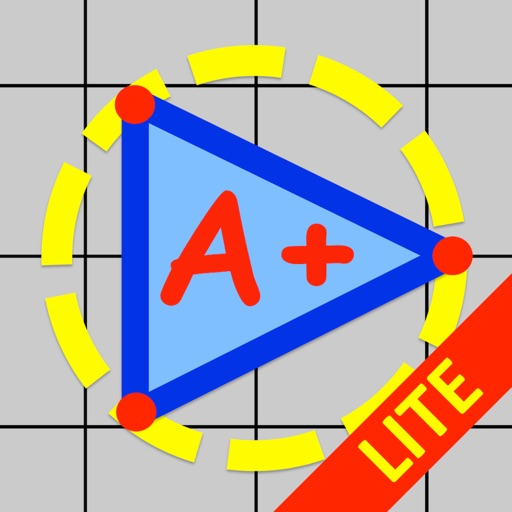## Geometry Ace is a fusion between a graphics based calculator and a geometry textbook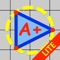# Geometry Ace Lite: Math Tutor

by Interactive Mindware LLC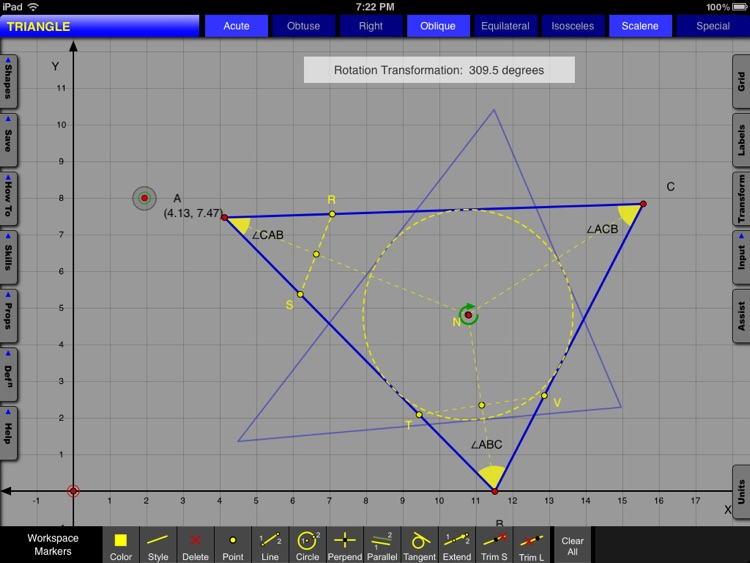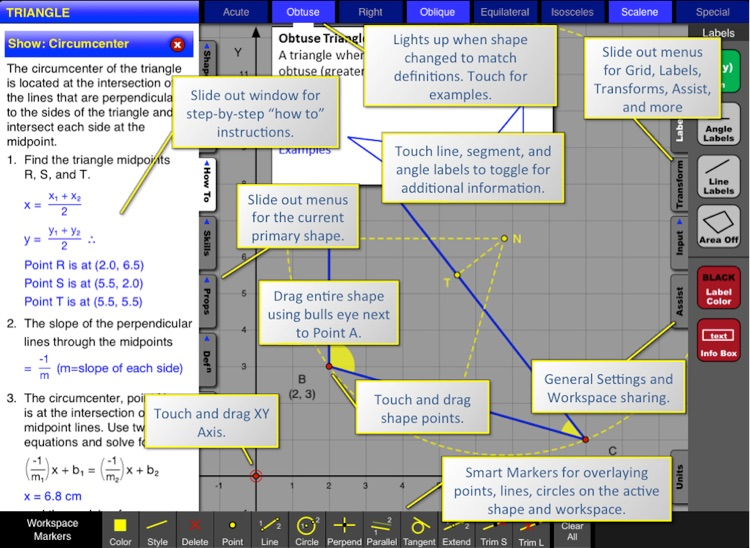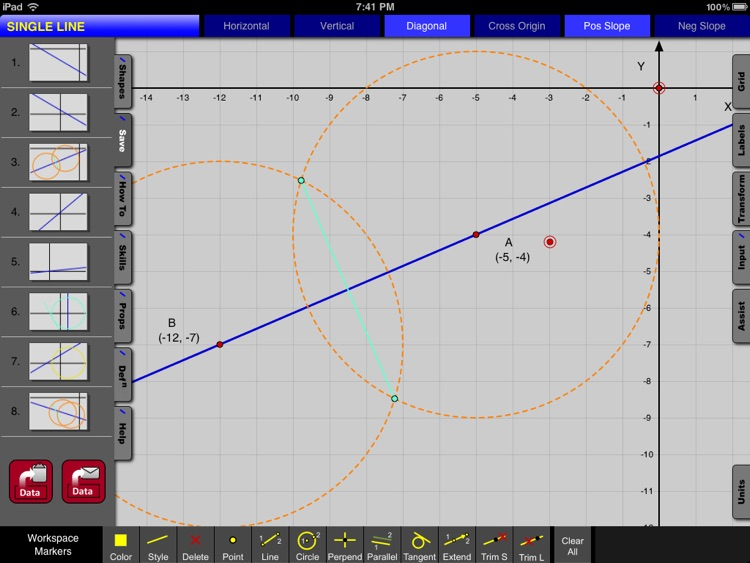Geometry Ace is a fusion between a graphics based calculator and a geometry textbook. Detailed step-by-step solutions update as you create new math problems by changing the graphics. Learn vocabulary with unlimited examples. Try before you buy! Full functionality for Points and Lines including transformations and constructions. Preview for Segments, Rays, Angles, Triangles, Quadrilaterals, Polygons, and Circles. Low cost in-app purchase upgrades to full function for all shapes. No ads!### App Details

Version
5.0.14
Rating
(2)
Size
28Mb
Genre
Education Reference
Last updated
February 26, 2018
Release date
May 2, 2015

• \$0.99

### App Screenshots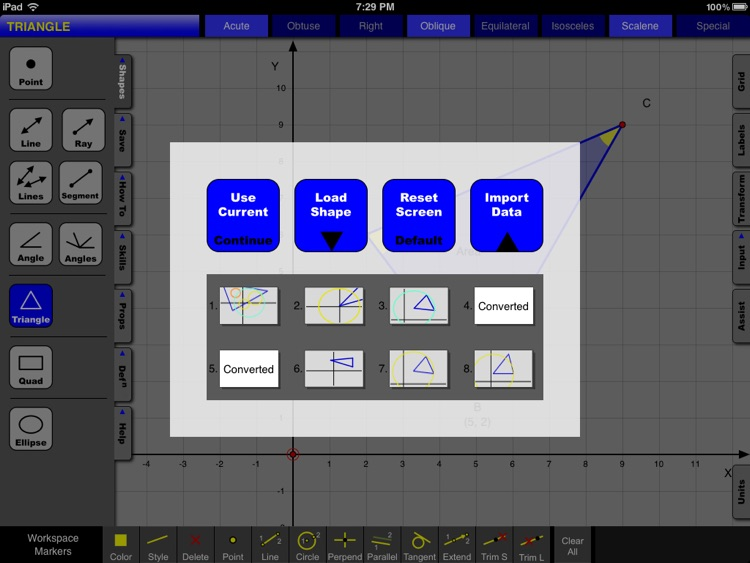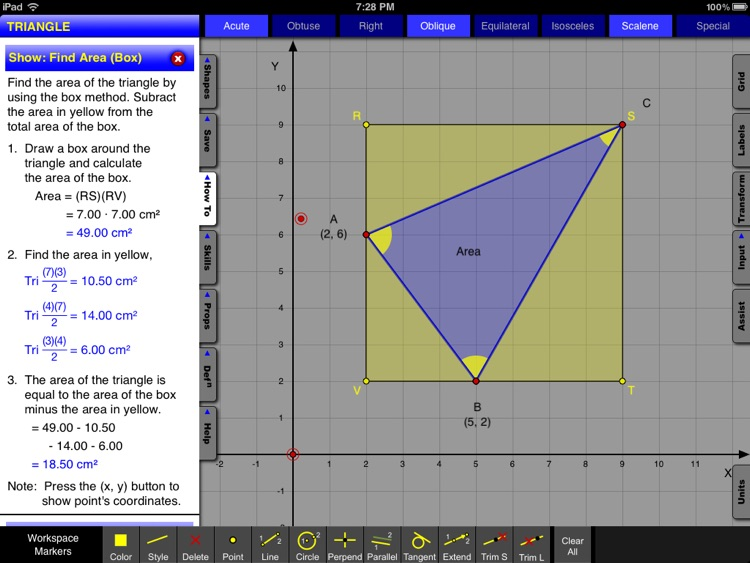### App Store Description

Geometry Ace is a fusion between a graphics based calculator and a geometry textbook. Detailed step-by-step solutions update as you create new math problems by changing the graphics. Learn vocabulary with unlimited examples. Try before you buy! Full functionality for Points and Lines including transformations and constructions. Preview for Segments, Rays, Angles, Triangles, Quadrilaterals, Polygons, and Circles. Low cost in-app purchase upgrades to full function for all shapes. No ads!

*** Easy Start-up ***
• Simply choose shape to study.
• Shape will already be on the screen and all you have to do is start manipulating it.
• Learn vocabulary, choose from list of tutorials, perform transformations, or create a construction.

*** Detailed problem solutions update as you change the graphics. ***
• You are not limited to a set of pre-written or randomly generated problems.
• Geometry Ace does not simply calculate lengths and areas for you. It shows you step-by-step how to do it!

*** Includes introductory material and makes connections with algebra. ***
• Introductory material appropriate for upper elementary students is included.
• Helps with differentiation. Middle and high school students who need a refresher can review introductory topics.
• Algebra topics needed for coordinate geometry are included.
• Introductory topics include coordinates of a point, finding slope, and writing the equation of a line.
• Answers to problems are given in fraction form when possible.
• Solutions address common student misconceptions and give attention to detail.

*** Interactive definitions ***
• Relevant definitions highlight interactively as you drag points and lines to change the shape on the screen to meet various attributes.
• Example: A triangle can be obtuse, oblique, and scalene.
• Example: A polygon can be concave and irregular.
• Definitions are inclusive. Example: A quadrilateral that is a rectangle is also a parallelogram and a trapezoid.

*** Transformations: Rotations, Reflections, and Translations ***
• Easily change degrees of rotation, center of rotation, line of reflection, and translation vector.
• Point mapping is shown!
• Perform successive transformations on the same object.

*** Constructions ***
• Includes points, lines, segments, circles, perpendicular lines, parallel lines, tangents, and angle bisectors.
• Easily make attachments to points, lines, circles, intersections, and midpoints.

*** Monitor Students ***
• Teachers can view student’s iPad screens who are on the same local wifi network and running this app.

TOPICS AVAILABLE:
Note: LT version has full function for points and lines only. Other shapes are in preview mode.

POINT:
Coordinates
Distance between points

LINE/RAY/SEGMENT:
Slope
Vertical, Horizontal
Y-intercept
Equation of line/ray/segment
Distance between point and line
Construct or write equation of parallel or perpendicular line
Segment midpoint and length
Construct or write equation of perpendicular bisector
Intersection point of 2 lines
Transversals
Alternate interior angles
Alternate exterior angles
Corresponding angles
Same side interior angles
Vertical angles

ANGLE:
Vertex, Legs, Interior, Measure
Acute, Right, Obtuse, Straight, Reflex
Construct or write equation for angle bisector
Congruent, Adjacent, Complimentary, Supplementary, Linear Pair, Vertical

TRIANGLE:
Acute, Obtuse, Right, Oblique, Equilateral, Isosceles, Scalene
Perimeter
Area – basic elementary
Area – coordinate plane - algebra
Area – box method
Centroid, Circumcenter, Incenter

Square, Rectangle, Rhombus, Parallelogram, Trapezoid, Kite
Perimeter
Area

POLYGON:
Triangle, Quadrilateral, Pentagon, Hexagon, Heptagon, Octagon, Nonagon, Decagon
Convex, Concave
Regular, Irregular
Sum of interior angles

CIRCLE: